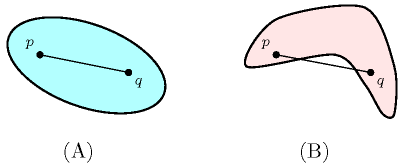### 3.7.62. Convex

A constraint involving the notion of convexity. A subset $𝒮$ of the plane is called convex if and only if for any pair of points $p,q$ of this subset the corresponding line segment is contained in $𝒮$. Part (A) of Figure 3.7.16 gives an example of convex set, while part (B) depicts an example of non-convex set.

##### Figure 3.7.16. (A) A convex set and (B) a non-convex set